• 对于二分类, F1 得分等于 Precision 与 Recall 的调和平均数, 公式如下: F1-Score=2∗Precsion∗RecallPrecision+Recall F1\text{-}Score=\frac{2*Precsion*Recall}{Precision+Recall} F1-Score=Precision+Recall2∗...
对于二分类, F1 得分等于 Precision 与 Recall 的调和平均数, 公式如下:

F

1

-

S

c

o

r

e

=

2

1

P

r

e

c

i

s

i

o

n

+

1

R

e

c

a

l

l

=

2

∗

P

r

e

c

s

i

o

n

∗

R

e

c

a

l

l

P

r

e

c

i

s

i

o

n

+

R

e

c

a

l

l

F1\text{-}Score=\frac{2}{\frac{1}{Precision}+\frac{1}{Recall}}=\frac{2*Precsion*Recall}{Precision+Recall}

对于多分类, 因为包含多个类别, F1 得分的计算包含 Macro-F1 和 Micro-F1 两种方式

创建示例数据
假设有 “A”, “B”, “C” 三个类别, 作了 16 次预测
import matplotlib.pyplot as plt
import numpy as np
import seaborn as sns
from sklearn.metrics import f1_score

# 3 种类别
CLASS_NAMES = ("A", "B", "C")

# 16 次预测
state = np.random.RandomState(seed=0)
# 实际标签
labels = state.randint(len(CLASS_NAMES), size=(16,))
# 预测标签
predicts = state.randint(len(CLASS_NAMES), size=(16,))

统计混淆矩阵
# 混淆矩阵
confusion = np.zeros(shape=(len(CLASS_NAMES), len(CLASS_NAMES)), dtype=np.int32)
for i in range(labels.shape):
confusion[labels[i], predicts[i]] += 1

# 打印混淆矩阵
print("混淆矩阵:", confusion, sep="\n")

结果:
混淆矩阵:
[[2 3 2]
[2 2 0]
[1 3 1]]

绘制混淆矩阵
# 绘制混淆矩阵
sns.set()

fig = plt.Figure(figsize=(6.4, 4.8))
ax: plt.Axes = fig.gca()
sns.heatmap(confusion, ax=ax, annot=True, cbar=True, fmt="d")
# X-轴与Y-轴 标签
ax.set_xlabel("Predict")
ax.set_ylabel("Actual")
# 刻度标签
ax.set_xticklabels(CLASS_NAMES)
ax.set_yticklabels(CLASS_NAMES)
# 保存可视化结果
fig.savefig("./confusion.png")

结果:样本统计(以 A 类别为例)
TP: 预测是 A 类, 实际上也是 A 类

FN: 预测不是 A 类, 实际上是 A 类

FP: 预测是 A 类, 实际上不是 A 类


A 类别
TPFNFP23+2 (第一行除去 A)2+1 (第一列除去 A)
B 类别
TPFNFP226
C 类别
TPFNFP142

Macro-F1
计算每一个类别的 Precision 和 Recall
Precision = TP / (TP + FP)
Recall = TP / (TP + FN)
A 类别:
Precision = 2 / (2+3) = 0.4
Recall = 2 / (2+5) = 0.286
B 类别:
Precision = 2 / (2+6) = 0.25
Recall = 2 / (2+2) = 0.5
C 类别:
Precision = 1 / (1+2) = 0.333
Recall = 1 / (1+4) = 0.2
# 准确率(多个类别)
class_p = confusion.diagonal() / confusion.sum(axis=0)
print("class_p:", class_p)
# 召回率(多个类别)
class_r = confusion.diagonal() / confusion.sum(axis=1)
print("class_r:", class_r)

结果
class_p: [0.4        0.25       0.33333333]
class_r: [0.28571429 0.5        0.2       ]


手动计算 Macro-F1
# ---------- 手动计算 Macro F1 ----------

# 防止分母为零
eps = 1e-6
# F1 得分(多个类别)
class_f1 = 2 * class_r * class_p / (class_r + class_p + eps)
print("各类别 F1 得分", class_f1)
# 各类别取平均值
macro_f1 = class_f1.mean()
print("Macro F1:", macro_f1)

各类别 F1 得分 [0.33333285 0.33333289 0.24999953]
Macro F1: 0.3055550891210972

sklearn 验证
print("Macro F1 in sklearn:", f1_score(labels, predicts, average="macro"))

Macro F1: 0.3055550891210972


Micro-F1
计算总体的 Precision 和 Recall, 由此得到总体的 F1-Score
总体样本的统计如下(将各个类别值相加):
TPFNFP51111
Precision = 5 / (5+11) = 0.3125
Recall = 5 / (5+11) = 0.3125
# ---------- 手动计算 Micro F1 ----------

# 准确率 = 召回率
p = r = confusion.diagonal().sum() / confusion.sum()
print("p or r:", p)
micro_f1 = 2 * r * p / (r + p)
print("Micro F1:", micro_f1)

结果
p or r: 0.3125
Micro F1: 0.3125

总体样本 Precision=Recall=F1-Score
sklearn 验证
print("Micro F1 in sklearn:", f1_score(labels, predicts, average="micro"))

Micro F1 in sklearn: 0.3125

展开全文机器学习
• 边界F1得分-Python实现 这是bfscore（用于图像分割的轮廓匹配分数）的开源python实现，用于多类图像分割，由SEOMULTECH的EMCOM LAB实现。 参考： 跑步 要运行该函数，只需在设置图像路径和阈值后运行python bfscore....
• 文章目录一、常用评估指标二、问题二、分析过程三、参考 ...F1得分：是调和平均的精确度和灵敏度 二、问题 二、分析过程 获取混淆矩阵 算法1: 算法2： 计算 算法1 查准率:P =0.975 查全率:R =0.9512 F1-s


文章目录
一、常用评估指标二、问题二、分析过程三、参考

一、常用评估指标
查全率: 真实正例被预测为正例的比例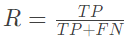真正例率: 真实正例被预测为正例的比例

显然查全率与真正例率是相等的。

查准率:预测为正例的实例中真实正例的比例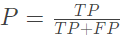假正例率: 真实反例被预测为正例的比例

两者并没有直接的数值关系。

F1得分：是调和平均的精确度和灵敏度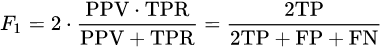二、问题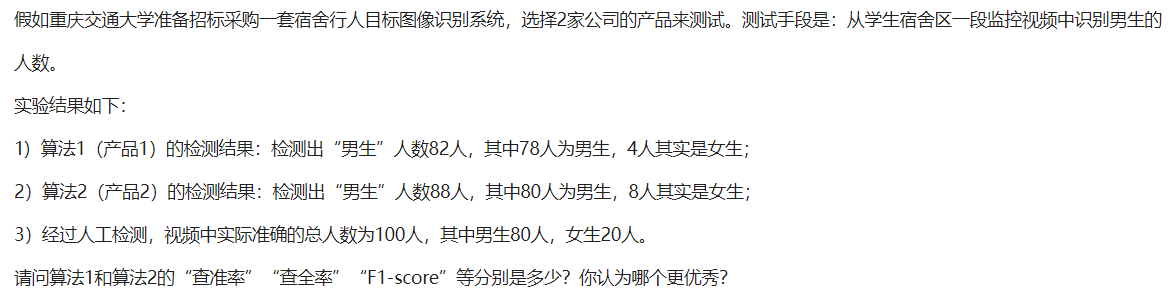二、分析过程
获取混淆矩阵 算法1:算法2：计算
算法1
查准率:P =0.975查全率:R =0.9512F1-score:F 1 =0.963
算法2
查准率:P = 1查全率:R = 0.91F1-score:F 1 = 0.952
评估
从查准率评价指标来看，算法2都要优于算法1，从查全率和F1度量评价指标来看，算法1都要优于算法2。总的来说，算法2更好
三、参考
【机器学习】(周志华–西瓜书) 真正例率（TPR）、假正例率（FPR）与查准率（P）、查全率（R）
展开全文•python 机器学习
• For example, a Precision of 0.01 and Recall of 1.0 would give : 这个想法是提供一个单一的指标，以平衡的方式对两个比率(精确度和召回率)进行加权，要求两者都具有较高的值才能使F1得分的值上升。 例如，...


Terminology of a specific domain is often difficult to start with. With a software engineering background, machine learning has many such terms that I find I need to remember to use the tools and read the articles.
特定领域的术语通常很难入手。 在软件工程的背景下，机器学习有许​​多这样的术语，我发现我需要记住要使用这些工具并阅读文章。
Some basic terms are Precision, Recall, and F1-Score. These relate to getting a finer-grained idea of how well a classifier is doing, as opposed to just looking at overall accuracy. Writing an explanation forces me to think it through, and helps me remember the topic myself. That’s why I like to write these articles.
一些基本术语是Precision，Recall和F1-Score。 这些与获得更精细的分类器效果的想法有关，而不是仅仅关注整体准确性。 撰写说明会迫使我仔细考虑，并帮助我自己记住该主题。 这就是为什么我喜欢写这些文章。
I am looking at a binary classifier in this article. The same concepts do apply more broadly, just require a bit more consideration on multi-class problems. But that is something to consider another time.
我正在看本文中的二进制分类器。 相同的概念的确适用范围更广，只需要对多类问题进行更多考虑即可。 但这是另一回事了。
Before going into the details, an overview figure is always nice:
在进入细节之前，总览图总是很不错的：
On the first look, it is a bit of a messy web. No need to worry about the details for now, but we can look back at this during the following sections when explaining the details from the bottom up. The metrics form a hierarchy starting with the the true/false negatives/positives (at the bottom), and building up all the way to the F1-score to bind them all together. Lets build up from there.
乍一看，它有点混乱。 现在无需担心细节，但是在从下至上解释细节时，我们可以在以下各节中回顾一下。 指标形成一个层次结构，从真/假否定/正数 (在底部)开始，并一直建立到F1分数以将它们全部绑定在一起。 让我们从那里开始。
正确/错误肯定和否定 (True/False Positives and Negatives)
A binary classifier can be viewed as classifying instances as positive or negative:
二进制分类器可以视为将实例分类为正数或负数：
Positive: The instance is classified as a member of the class the classifier is trying to identify. For example, a classifier looking for cat photos would classify photos with cats as positive (when correct). 肯定的 ：实例被分类为分类器试图识别的类的成员。 例如，寻找猫照片的分类器会将猫的照片分类为正(正确时)。 Negative: The instance is classified as not being a member of the class we are trying to identify. For example, a classifier looking for cat photos should classify photos with dogs (and no cats) as negative. 负数 ：实例被归类为不是我们试图识别的类的成员。 例如，寻找猫照片的分类器应将带有狗(而不是猫)的照片分类为负。
The basis of precision, recall, and F1-Score comes from the concepts of True Positive, True Negative, False Positive, and False Negative. The following table illustrates these (consider value 1 to be a positive prediction):
精度，召回率和F1-Score的基础来自“ 真肯定” ，“ 真否定” ，“ 假肯定 ”和“ 假否定”的概念 。 下表对此进行了说明(将值1视为肯定预测)：

Examples of True/False Positive and Negative

正/负正负示例

真正(TP) (True Positive (TP))
The following table shows 3 examples of a True Positive (TP). The first row is a generic example, where 1 represents the Positive prediction. The following two rows are examples with labels. Internally, the algorithms would use the 1/0 representation, but I used labels here for a more intuitive understanding.
下表显示了3个正正(TP)示例。 第一行是一般示例，其中1表示肯定预测。 以下两行是带有标签的示例。 在内部，算法将使用1/0表示形式，但是我在这里使用标签是为了更直观地理解。

Examples of True Positive (TP) relations.

真正(TP)关系的示例。

误报(FP) (False Positive (FP))
These False Positives (FP) examples illustrate making wrong predictions, predicting Positive samples for a actual Negative samples. Such failed prediction is called False Positive.
这些误报(FP)示例说明了错误的预测，为实际的负样本预测了正样本。 这种失败的预测称为误报。

真负(TN) (True Negative (TN))
For the True Negative (TN) example, the cat classifier correctly identifies a photo as not having a cat in it, and the medical image as the patient having no cancer. So the prediction is Negative and correct (True).
对于True Negative(TN)示例，猫分类器正确地将照片识别为其中没有猫，而医学图像则将其识别为没有癌症的患者。 因此，该预测为负且正确(正确)。

假阴性(FN) (False Negative (FN))
In the False Negative (FN) case, the classifier has predicted a Negative result, while the actual result was positive. Like no cat when there is a cat. So the prediction was Negative and wrong (False). Thus it is a False Negative.
在假阴性(FN)情况下，分类器预测的结果为阴性，而实际结果为肯定。 就像没有猫的猫一样。 因此，该预测是负面的和错误的(错误)。 因此，这是一个假阴性。

混淆矩阵 (Confusion Matrix)
A confusion matrix is sometimes used to illustrate classifier performance based on the above four values (TP, FP, TN, FN). These are plotted against each other to show a confusion matrix:
有时会使用混淆矩阵来说明基于上述四个值(TP，FP，TN，FN)的分类器性能。 这些相互绘制以显示混淆矩阵：
Confusion Matrix.

Using the cancer prediction example, a confusion matrix for 100 patients might look something like this:
使用癌症预测示例，一个100位患者的混淆矩阵可能看起来像这样：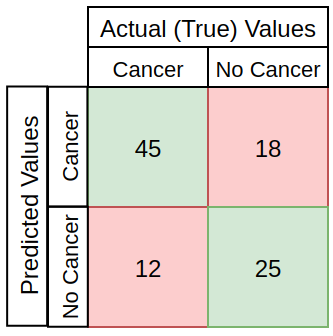This example has:
这个例子有：
TP: 45 positive cases correctly predicted TP：正确预测45例阳性病例 TN: 25 negative cases correctly predicted TN：正确预测25例阴性病例 FP: 18 negative cases are misclassified (wrong positive predictions) FP：18例阴性病例被错误分类(错误的阳性预测) FN: 12 positive cases are misclassified (wrong negative predictions) FN：12个阳性病例被错误分类(错误的阴性预测)
Thinking about this for a while, there are different severities to the different errors here. Classifying someone who has cancer as not having it (false negative, denying treatment), is likely more severe than classifying someone who does not have it as having it (false positive, consider treatment, do further tests).
考虑一段时间，这里的不同错误有不同的严重程度。 将患有癌症的人分类为没有癌症(假阴性，拒绝治疗)可能比将没有癌症的人分类为癌症(假阳性，考虑治疗，做进一步的检查)更为严格。
As the severity of different kinds of mistakes varies across use cases, the metrics such as Accuracy, Precision, Recall, and F1-score can be used to balance the classifier estimates as preferred.
由于不同类型错误的严重性随使用案例的不同而不同，因此可以使用诸如Accuracy ， Precision ， Recall和F1分数之类的指标来平衡分类器估计值。
准确性 (Accuracy)
The base metric used for model evaluation is often Accuracy, describing the number of correct predictions over all predictions:
用于模型评估的基本度量通常是Accuracy ，它描述了所有预测中正确预测的数量：
Accuracy Formulas.

These three show the same formula for calculating accuracy, but in different wording. From more formalized to more intuitive (my opinion). In the above cancer example, the accuracy would be:
这三个显示相同的公式以计算准确性，但是用不同的措词。 从更形式化到更直观(我认为)。 在上述癌症示例中，准确性为：
(TP+TN)/DatasetSize=(45+25)/100=0.7=70%. (TP + TN)/数据集大小=(45 + 25)/100=0.7=70%。
This is perhaps the most intuitive of the model evaluation metrics, and thus commonly used. But often it is useful to also look a bit deeper.
这也许是模型评估指标中最直观的，因此是常用的。 但是通常看起来更深也很有用。
精度/灵敏度 (Precision / Sensitivity)
Precision is a measure of how many of the positive predictions made are correct (true positives). It is sometimes also referred to as Sensitivity. The formula for it is:
精度是对做出的肯定预测中有多少是正确的(真实肯定)的度量。 有时也称为敏感度 。 公式为：
Precision formulas.

All three above are again just different wordings of the same, with the last one using the cancer case as a concrete example. In this cancer example, using the values from the above example confusion matrix, the precision would be:
上面的所有三个都只是相同的不同用语，最后一个以癌症病例为例。 在这个癌症示例中，使用上述示例混淆矩阵中的值，精度为：
45/(45+18)=45/63=0.714=71.4%. 45 /(45 + 18)= 45/63 = 0.714 = 71.4％。
召回 (Recall)
Recall is a measure of how many of the positive cases the classifier correctly predicted, over all the positive cases in the data. The formula for it is:
回忆是衡量分类器在数据中所有阳性病例中正确预测的阳性病例数的一种度量。 公式为：
Recall formulas.

Once again, this is just the same formula worded three different ways. For the cancer example, using the confusion matrix data, the recall would be:
再一次，这只是用三个不同方式措辞的相同公式。 对于癌症示例，使用混淆矩阵数据，召回将是：
45/(45+12)=45/57=0.789=78.9%. 45 /(45 + 12)= 45/57 = 0.789 = 78.9％。
特异性 (Specificity)
Specificity is a measure of how many negative predictions made are correct (true negatives). The formula for it is:
特异性是衡量做出的阴性预测是正确的(真实阴性)的量度。 公式为：
Specificity formulas.

In the above medical example, the specificity would be:
在上述医学示例中，特异性为：
25/(25+18)=0.581=58,1%. 25 /(25 + 18)= 0.581 = 58,1％。
F1-分数 (F1-Score)
F1-Score is a measure combining both precision and recall. It is generally described as the harmonic mean of the two. Harmonic mean is just another way to calculate an “average” of values, generally described as more suitable for ratios (such as precision and recall) than the traditional arithmetic mean. The formula used for F1-score in this case is:
F1-分数是一种结合了精确度和召回率的度量。 通常将其描述为两者的谐波均值 。 谐波均值是计算值“平均值”的另一种方法，通常将其描述为比传统算术均值更适合比率(例如精度和查全率)。 在这种情况下，用于F1分数的公式为：
F1-Score formula.

The idea is to provide a single metric that weights the two ratios (precision and recall) in a balanced way, requiring both to have a higher value for the F1-score value to rise. For example, a Precision of 0.01 and Recall of 1.0 would give :
这个想法是提供一个单一的指标，以平衡的方式对两个比率(精确度和召回率)进行加权，要求两者都具有较高的值才能使F1得分的值上升。 例如，Precision为0.01，Recall为1.0会得出：
an arithmetic mean of (0.01+1.0)/2=0.505, (0.01 + 1.0)/2=0.505的算术平均值， F1-score score (formula above) of 2*(0.01*1.0)/(0.01+1.0)=~0.02. F1得分2 *(0.01 * 1.0)/(0.01 + 1.0)=〜0.02。
This is because the F1-score is much more sensitive to one of the two inputs having a low value (0.01 here). Which makes it great if you want to balance the two.
这是因为F1分数对具有低值(此处为0.01)的两个输入之一更为敏感。 如果您想平衡两者，那就太好了。
F1分数的一些优点：
Very small precision or recall will result in lower overall score. Thus it helps balance the two metrics. 极小的精度或召回率将导致较低的总体得分。 因此，它有助于平衡两个指标。 If you choose your positive class as the one with fewer samples, F1-score can help balance the metric across positive/negative samples. 如果您选择阳性类别作为样本较少的类别，则F1得分可以帮助在阳性/阴性样本之间平衡指标。 As illustrated by the first figure in this article, it combines many of the other metrics into a single one, capturing many aspects at once. 如本文第一个图所示，它将许多其他指标合并为一个指标，一次捕获了许多方面。
In the cancer example further above, the F1-score would be
在上面的癌症示例中，F1得分为
2 * (0.714*0.789)/(0.714+0.789)=0.75 = 75% 2 *(0.714 * 0.789)/(0.714 + 0.789)= 0.75 = 75％
探索F1分数 (Exploring F1-score)
I find it easiest to understand concepts by looking at some examples. First a function in Python to calculate F1-score:
通过查看一些示例，我发现最容易理解概念。 首先在Python中使用一个函数来计算F1得分：
Python implementation of the F1-score formula.

To compare different combinations of precision and recall, I generate example values for precision and recall in range of 0 to 1 with steps of 0.01 (100 values of 0.01, 0.02, 0.03, … , 1.0):
为了比较精度和查全率的不同组合，我生成了精度和查全率的示例值，范围为0至1，步距为0.01(100个值分别为0.01、0.02、0.03，…，1.0)：
Generating example values for precision and recall.

This produces a list for both precision and recall to experiment with:
这会产生精度和召回率的列表，供您尝试：
Generated precision and recall values.

精度=召回时为F1得分 (F1-score when precision=recall)
To see what is the F1-score if precision equals recall, we can calculate F1-scores for each point 0.01 to 1.0, with precision = recall at each point:
要查看精度等于召回率的F1分数，我们可以计算0.01至1.0的每个点的F1分数，其中精度=召回率：
Calculating F1-Score for the example values, where precision = recall at each 100 points.

F1-score when precision = recall. F1-score equals precision and recall at each point when p=r.

F1-score equals precision and recall if the two input metrics (P&R) are equal. The Difference column in the table shows the difference between the smaller value (Precision/Recall) and F1-score. Here they are equal, so no difference, in following examples they start to vary.
如果两个输入指标(P＆R)相等，则F1分数等于精度和召回率。 表格中的“ 差异”列显示较小的值(“精确度/调用”)与F1分数之间的差异。 在这里，它们是相等的，因此没有区别，在以下示例中，它们开始变化。
回忆= 1.0，精度= 0.01至1.0时为F1分数 (F1-score when Recall = 1.0, Precision = 0.01 to 1.0)
So, the F1-score should handle reasonably well cases where one of the inputs (P/R) is low, even if the other is very high.
因此，F1分数应该可以很好地处理其中一个输入(P / R)为低，即使另一个非常高的情况。
Lets try setting Recall to the maximum of 1.0 and varying Precision from 0.01 to 1.0:
让我们尝试将Recall设置为最大值1.0，并将Precision从0.01更改为1.0：
Calculating F1-Score when recall is always 1.0 and precision varies from 0.01 to 1.0.

F1-score when recall = 1.0 and precision varies from 0.1 to 1.0.

As expected, the F1-score stays low when one of the two inputs (Precision / Recall) is low. The difference column shows how the F1-score in this case rises a bit faster than the smaller input (Precision here), gaining more towards the middle of the chart, weighted up a bit by the bigger value (Recall here). However, it never goes very far from the smaller input, balancing the overall score based on both inputs. These differences can also be visualized on the figure (difference is biggest at the vertical red line):
不出所料，当两个输入之一( Precision / R ecall)为低时，F1分数保持为低。 差异列显示了这种情况下F1得分的上升速度比较小的输入(此处为Precision )如何更快，在图表的中间位置获得了更多收益，由较大的值进行了加权(此处为Recall )。 但是，它与较小的输入绝不会相差很远，可以基于两个输入来平衡总分。 这些差异也可以在图形上看到( 差异在垂直红线处最大)：
F1-Score with precision = 1.0, recall = 0–1.0 with highlighted posts.

当Precision = 1.0和Recall = 0.01至1.0时为F1分数 (F1-score when Precision = 1.0 and Recall = 0.01 to 1.0)
If we swap the roles of Precision and Recall in the above example, we get the same result (due to F1-score formula):
如果在上面的示例中互换Precision和Recall的角色，我们将得到相同的结果(由于F1得分公式)：
Calculating F1-Score when precision is always 1.0 and recall varies from 0.0 to 1.0.

F1-score when precision = 1.0 and recall varies from 0.01 to 1.0.

This is to say, regardless of which one is higher or lower, the overall F1-score is impacted in the exact same way (which seems quite obvious in the formula but easy to forget).
也就是说，无论哪个F1分数更高或更低，都会以完全相同的方式影响整个F1分数 (这在公式中似乎很明显，但很容易忘记)。
当Precision = 0.8且Recall = 0.01至1.0时为F1分数 (F1-score when Precision=0.8 and Recall = 0.01 to 1.0)
Besides fixing one input at maximum, lets try a bit lower. Here precision is fixed at 0.8, while Recall varies from 0.01 to 1.0 as before:
除了最大固定一个输入外，让我们尝试降低一点。 这里的精度固定为0.8，而调用的范围从0.01到1.0像以前一样：
Calculating F1-Score when precision is always 0.8 and recall varies from 0.0 to 1.0.

F1-score when precision = 0.8 and recall varies from 0.01 to 1.0.

The top score with inputs (0.8, 1.0) is 0.89. The rising curve shape is similar as Recall value rises. At maximum of Precision = 1.0, it achieves a value of about 0.1 (or 0.09) higher than the smaller value (0.89 vs 0.8).
输入(0.8，1.0)的最高得分是0.89。 曲线上升的形状类似于召回值的上升。 在Precision = 1.0的最大值下，其值比较小的值(0.89对0.8)高约0.1(或0.09)。
当Precision = 0.1和Recall = 0.01至1.0时为F1分数 (F1-score when Precision=0.1 and Recall=0.01 to 1.0)
And if we fix one value near minimum at 0.1?
如果我们将一个值固定在最小值0.1附近？
Calculating F1-Score when precision is always 0.1 and recall varies from 0.0 to 1.0.

F1-score when precision = 0.1 and recall varies from 0.01 to 1.0.

Because one of the two inputs is always low (0.1), the F1-score never rises very high. However, interestingly it again rises at maximum to about 0.08 value larger than the smaller input (Precision = 0.1, F1-score=0.18). This is quite similar to the fixed value of Precision = 0.8 above, where the maximum value reached was 0.09 higher than the smaller input.
因为两个输入之一始终为低(0.1)，所以F1分数永远不会升高到很高。 然而，有趣的是，它再次最大上升到比较小输入更大的约0.08值( 精度 = 0.1， F1分数 = 0.18)。 这与上面的Precision = 0.8的固定值非常相似，其中达到的最大值比较小的输入高0.09。
将F1得分集中在精度或召回率上 (Focusing F1-score on precision or recall)
Besides the plain F1-score, there is a more generic version, called Fbeta-score. F1-score is a special instance of Fbeta-score, where beta=1. It allows one to weight the precision or recall more, by adding a weighting factor. I will not go deeper into that in this post, however, it is something to keep in mind.
除了普通的F1-score之外 ，还有一个更通用的版本，称为Fbeta-score 。 F1分数是Fbeta 分数的特殊实例，其中beta = 1。 通过添加加权因子，它可以使精度加权或提高查全率。 在这篇文章中，我不会对此做更深入的介绍，但是要牢记这一点。
F1得分vs准确性 (F1-score vs Accuracy)
Accuracy is commonly described as a more intuitive metric, with F1-score better addressing a more imbalanced dataset. So how does the F1-score (F1) vs Accuracy (ACC) compare across different types of data distributions (ratios of positive/negative)?
准确性通常被描述为一种更直观的度量标准， F1分数可以更好地解决数据集更加不平衡的问题。 那么，如何在不同类型的数据分布(正/负比率)之间比较F1分数 ( F1 )与准确性 ( ACC )？
失衡：很少有积极案例 (Imbalance: Few Positive Cases)
In this example, there is an imbalance of 10 positive cases, and 90 negative cases, with different TN, TP, FN, and FP values for a classifier to calculate F1 and ACC:
在此示例中，存在10个阳性案例和90个阴性案例的不平衡，其中TN，TP，FN和FP值不同，用于分类器来计算F1和ACC：
F1-score vs accuracy with varying prediction rates and imbalanced data.

The maximum accuracy with the class imbalance is with a result of TN=90 and TP=10, as shown on row 2.
类不平衡的最大精度是TN = 90和TP = 10，如第2行所示。
In each case where TP =0, the Precision and Recall both become 0, and F1-score cannot be calculated (division by 0). Such cases can be scored as F1-score = 0, or generally marking the classifier as useless. Because the classifier cannot predict any correct positive result. This is rows 0, 4, and 8 in the above table. These also illustrate some cases of high Accuracy for a broken classifier (e.g., row 0 with 90% Accuracy while always predicting only negative).
在TP = 0的每种情况下， Precision和Recall都变为0，并且无法计算F1得分 (除以0)。 可以将此类情况评分为F1分数= 0，或者通常将分类器标记为无用。 因为分类器无法预测任何正确的阳性结果。 这是上表中的第0、4和8行。 这些也说明高准确度的某些情况下，一个破碎分类器(例如，具有90％的准确度的行0而总是预测仅负)。
The remaining rows illustrate how the F1-score is reacting much better to the classifier making more balanced predictions. For example, F1-score=0.18 vs Accuracy = 0.91 on row 5, to F1-score=0.46 vs Accuracy = 0.93 on row 7. This is only a change of 2 positive predictions, but as it is out of 10 possible, the change is actually quite large, and the F1-score emphasizes this (and Accuracy sees no difference to any other values).
其余各行说明F1分数对分类器做出更好的React如何做出更平衡的预测。 例如，第5行的F1得分 = 0.18 vs 准确度 = 0.91，第7行的F1得分 = 0.46 vs 准确度 = 0.93。这只是2个肯定预测的变化，但是由于它在10个可能的结果中，变化实际上很大， F1分数强调了这一点(“ 精度”与其他任何值都没有区别)。
平衡50/50阳性和阴性病例： (Balance 50/50 Positive and Negative cases:)
How about when the datasets are more balanced? Here are similar values for a balanced dataset with 50 negative and 50 positive items:
数据集何时更平衡呢？ 以下是具有50个负数和50个正数项的平衡数据集的相似值：
F1-score vs accuracy with varying prediction rates and balanced data.

F1-score is still a slightly better metric here, when there are only very few (or none) of the positive predictions. But the difference is not as huge as with imbalanced classes. In general, it is still always useful to look a bit deeper into the results, although in balanced datasets, a high accuracy is usually a good indicator of a decent classifier performance.
当只有很少(或没有)肯定预测时， F1分数仍然是一个更好的指标。 但是区别并不像不平衡的班级那样巨大。 通常，深入了解结果仍然总是有用的，尽管在平衡数据集中，高精度通常是良好的分类器性能的良好指标。
失衡：很少有负面案例 (Imbalance: Few Negative Cases)
Finally, what happens if the minority class is measured as the negative and not positive? F1-score no longer balances it but rather the opposite. Here is an example with 10 negative cases and 90 positive cases:
最后，如果将少数群体视为消极而不是积极的话会怎样？ F1分数不再平衡，而是相反。 这是一个带有10个否定案例和90个肯定案例的示例：
F1-score vs Accuracy when the positive class is the majority class.

For example, row 5 has only 1 correct prediction out of 10 negative cases. But the F1-score is still at around 95%, so very good and even higher than accuracy. In the case where the same ratio applied to the positive cases being the minority, the F1-score for this was 0.18 vs now it is 0.95. Which was a much better indicator of quality rather than in this case.
例如，第5行在10个否定情况下只有1个正确的预测。 但是F1分数仍然在95％左右，因此非常好，甚至比准确性更高。 在相同的比例适用于阳性病例为少数的情况下， F1评分为0.18，而现在为0.95。 比起这种情况，这是更好的质量指标。
This result with minority negative cases is because of how the formula to calculate F1-score is defined over precision and recall (emphasizing positive cases). If you look back at the figure illustrating the metrics hierarchy at the beginning of this article, you will see how True Positives feed into both Precision and Recall, and from there to F1-score. The same figure also shows how True Negatives do not contribute to F1-score at all. This seems to be viisble here if you reverse the ratios and have fewer true negatives.
少数否定案例的结果是因为如何在精确度和召回率 (强调肯定案例)上定义了计算F1分数的公式。 如果您回顾一下本文开头说明指标层次结构的图，您将看到True Positives如何馈入Precision和Recall ，并从那里馈入F1评分 。 同一张图还显示了真否定词根本对F1分数没有贡献。 如果您反转比率并减少真实负数，那么这似乎是可行的。
So, as usual, I believe it is good to keep in mind how to represent your data, and do your own data exploration, not blindly trusting any single metric.
因此，与往常一样，我认为最好记住如何表示您的数据并进行自己的数据探索，而不是盲目地信任任何单一指标。
结论 (Conclusions)
So what are these metrics good for?
那么这些指标有什么好处呢？
The traditional Accuracy is a good measure if you have quite balanced datasets and are interested in all types of outputs equally. I like to start with it in any case, as it is intuitive, and dig deeper from there as needed.
如果您有相当平衡的数据集并且对所有类型的输出都同样感兴趣，那么传统的准确性是一个很好的衡量标准。 在任何情况下，我都喜欢从它开始，因为它很直观，并且可以根据需要从那里进行更深入的研究。
Precision is great to focus on if you want to minimize false positives. For example, you build a spam email classifier. You want to see as little spam as possible. But you do not want to miss any important, non-spam emails. In such cases, you may wish to aim for maximizing precision.
如果要最大程度地减少误报，可以将重点放在精确度上。 例如，您构建垃圾邮件分类器。 您希望看到的垃圾邮件越少越好。 但是您不想错过任何重要的非垃圾邮件。 在这种情况下，您可能希望最大程度地提高精度。
Recall is very important in domains such as medical (e.g., identifying cancer), where you really want to minimize the chance of missing positive cases (predicting false negatives). These are typically cases where missing a positive case has a much bigger cost than wrongly classifying something as positive.
召回在医疗(例如，识别癌症)等领域非常重要，在该领域中，您确实希望最大程度地减少漏报阳性病例(预测假阴性)的机会。 在这些典型情况下，错过一个肯定的案例所付出的代价要比错误地将某事物归类为肯定的情况要大得多。
Neither precision nor recall is necessarily useful alone, since we rather generally are interested in the overall picture. Accuracy is always good to check as one option. F1-score is another.
精确度和召回率都不一定有用，因为我们通常对整体情况感兴趣。 准确性始终是一个很好的选择。 F1分数是另一个。
F1-score combines precision and recall, and works also for cases where the datasets are imbalanced as it requires both precision and recall to have a reasonable value, as demonstrated by the experiments I showed in this post. Even if you have a small number of positive cases vs negative cases, the formula will weight the metric value down if the precision or recall of the positive class is low.
F1分数结合了精确度和查全率，并且也适用于数据集不平衡的情况，因为它要求精确度和查全率都具有合理的值，正如我在本文中展示的实验所证明的那样。 即使肯定案例与否定案例的数量很少，但如果肯定类的精度或召回率较低，该公式也会降低度量值的权重。
Besides these, there are various other metrics and ways to explore your results. A popular and very useful approach is also use of ROC- and precision-recall curves. These allow fine-tuning the evaluation thresholds according to what type of error we want to minimize. But that is a different topic to explore.
除了这些，还有其他各种指标和方法可以探索您的结果。 一种流行且非常有用的方法是使用ROC和精确调用曲线 。 这些允许根据我们要最小化的错误类型微调评估阈值。 但这是一个不同的主题。
Thats all for today.. :)
今天就这些了.. :)

翻译自: https://medium.com/@tkanstren/a-look-at-precision-recall-and-f1-score-36b5fd0dd3ec


展开全文•机器学习 python
• MachineLearning_Project 通过操纵神经网络的各种参数，已经开发出用于人类... 通过由2个卷积，2个最大池和2个线性层组成的体系结构，我们实现了96％的精度和90.52％的F1得分，优于在同一数据集上训练的几个最新模型。
• 安然欺诈项目 休斯顿的安然综合体- 安然是美国最大的公司之一。 由于公司欺诈，它破产了。 由于联邦调查的结果，大量的安然数据（电子邮件和财务数据）已进入公共记录。 该项目旨在建立一个分类器，该分类器可以...
• 最近在复盘udacity 的机器学习的课程，现在来整理一下关于机器学习模型的评估指标的相关知识。...学习模型的评估指标常用的有几种：F-β得分（F1得分，F2得分等等，根据不同的业务实际需求来指定适合的β值），ROC...机器学习 评估指标 精度 召回率
• 该模型通过两种方式进行训练：将经典的“二进制交叉熵”损失与旨在直接优化“宏观F1得分”的自定义“宏观软F1”损失进行比较。 第二种方法的好处被证明是非常有趣的。 请检查以下两个博客文章以获取完整描述： ...
• ## F1分数

千次阅读 2017-05-10 00:33:13
F1分数：既然已经讨论了precise（精确率）recall（回召率），接下来将使用一个新的机器学习指标F1分数，F1分数会同时考虑精确率和回召率，以便重新计算新的分数。F1分数可以理解为：精确率和召回率的加权平均值。...
• 精准率和召回率3.F1值4.ROC AUC 一、垃圾短信分类器 对于垃圾短信分类器，当分类器将一条短信正确地预测为垃圾短信时为真阳性；当分类器将一条短信正确地预测为非垃圾短信时为真阴性；当非垃圾信息被预测为垃圾信息...
• F1 分数会同时考虑精确率和召回率，以便计算新的分数。 可将 F1 分数理解为精确率和召回率的加权平均值，其中 F1 分数的最佳值为 1、最差值为 0： F1 = 2 * (精确率 * 召回率) / (精确率 + 召回率) 帮助文档 ...
• F1-Score相关概念 F1分数（F1 Score），是统计学中用来衡量二分类（或多任务二分类）模型精确度的一种指标。它同时兼顾了分类模型的准确率和召回率。F1分数可以看作是模型准确率和召回率的一种加权平均，它的最大...机器学习 深度学习 二分类
• ## 主成分得分和因子得分

万次阅读 多人点赞 2020-05-03 14:44:37
记录一下主成分得分和因子得分 本文是基于各全国各省经济发展情况综合评价 首先贴上总得方差解释 A.成分矩阵 特别注意： 该成分矩阵（因子载荷矩阵）并不是主成分的特征向量，即不是主成分的系数。主成分系数的求...机器学习 数据分析
• 本文以我自己的实验数据来作为样例说明何为macro F1与micro F1 目录1 前置知识2 macro-F1与micro-F12.1 使用场景2.2 计算方法3 总结4 参考 1 前置知识 关于F1 score的内容如果还不清楚的朋友，可以参考我的上一篇...机器学习
• 在利用K_means 聚类、LR、SVM 分类，评估Embedding 结果的好坏时，遇到如下代码，不理解当中 micro_f1，macro_f1 的含义，开此篇学习记录。 def classification(x, y, method='XGBoost'): x_train, x_valid...评估指标
• 例如依照最上面的表格来计算:Precison=5/(5+4)=0.556,Recall=5/(5+4)=0.556，然后带入F1的公式求出F1，这种方式被称为Micro-F1微平均。 第二种方式是计算出每一个类的Precison和Recall后计算F1，最后将F1平均。 ...Recall Accuracy
• 分类是机器学习中比较常见的任务，对于分类任务常见的评价指标有准确率（Accuracy）、精确率（Precision）、召回率（Recall）、F1 score、ROC曲线（Receiver Operating Characteristic Curve）等。 这篇文章将结合...分类评估指标 混淆矩阵 Recall
• 对于多标签问题，引入Micro-F1和Macro-F1，以下对Micro-F1和Macro-F1的计算全已三个类别ABC为例： Macro-F1 M a c r o _ P = P A + P B + P C 3   M a c r o _ R = R A + R B + R C 3   M a c r o _ F 1 = 2 M a ...机器学习
• 在锦标赛结束时，得分最多的车手是冠军。如果有平分，则冠军是赢的最多的人（即排位第一）。如果还是平分，则选择得到排位第二最多的人，依此类推，直到没有更多的排位进行比较。 后来又提出了另一个得分制度，其中...
• 在锦标赛结束时，得分最多的车手是冠军。如果有平分，则冠军是赢的最多的人（即排位第一）。如果还是平分，则选择得到排位第二最多的人，依此类推，直到没有更多的排位进行比较。 后来又提出了另一个得分制度，其中...c++...